Tuesday, 14 July 2009

The geometry of Time and the broken symmetry of spacetime

Video The Symmetry of Time

~Two light sphere distorting the symmetry of spacetime

Within our Universe we see patterns of a beautiful symmetry both in nature and in mathematics. The same basic method of pattern formation can be seen in all living systems and nonliving ones nature’s symmetry can be found on every level of creation from spiral galaxies to sea shells from the rings of Saturn to living cells. There has to be a general principle underlying such universal symmetry. Could the imperfect symmetry that we observe in nature just be the broken traces of a far greater universal symmetry of our Universe?

In Quantum Atom Theory this universal symmetry is the geometry of time itself creating Einstein’s curvature of spacetime at the quantum level of the atoms. Because it is impossible to achieve absolute zero every atom is radiating EMR continuously in light spheres of quantized wave fronts creating the forward momentum of time.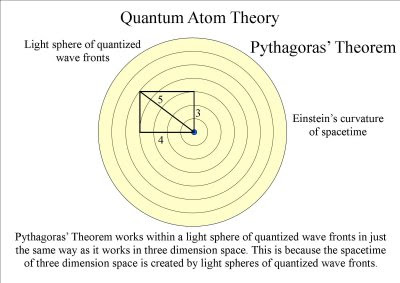The Pythagoras's' Theorem works within a light sphere of quantized wave fronts in just the same way as it works in three dimensional space. This is because the spacetime of three dimensional space is created light sphere of quantized wave fronts.The atoms will distort the geometry of time creating visual and mathematical patterns.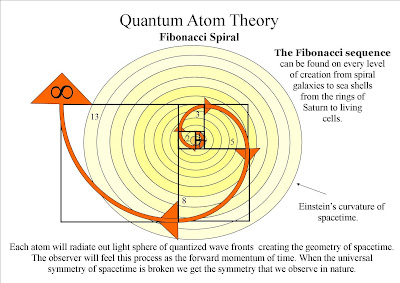Only a slight distortion in the spacetime symmetry will expand out creating the visual and mathematical pattern of our Universe.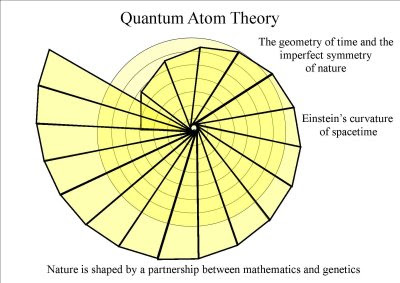Therefore we have a universal dynamically evolving geometry of time in the form of ever expanding spherical quantized wave fronts. The observer will feel this as the forward momentum of time and will see patterns of a beautiful symmetry not just because of their mathematical position in space but it is also because of their sequence in time.The atoms will distort the geometry of time creating mathematical patterns of every conceivable shape. The same basic method of pattern formation, the same mechanism of symmetry breaking governs the whole universe of organic and non organic matter. Everything will form into ever greater complexity because everything is creating its own spacetime geometry of ever greater diversity. This can explain the dynamics of evolution; from simple to complex

~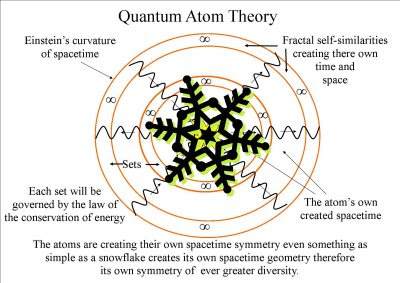The atoms of each snowflake are creating their own spacetime symmetry therefore their own spacetime geometry of ever greater beauty
~

Monday, 6 July 2009

Video on Quantum Gravity

Video on Quantum Gravity.
~

This video explains a new theory on the gravitational force uniting quantum mechanics and the classical mechanics of Newton.

~In Newton's third law of motion "to every action there is an equal and opposite reaction" in Quantum Atom Theory gravity is the opposite reaction to the atoms radiating quantized wave fronts of electromagnetic radiation. Most of this EMR is invisible to us but we will feel it as the forward momentum of time.

~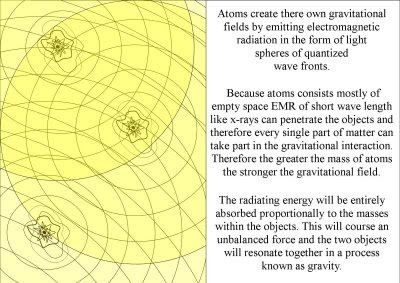Atoms create there own gravitational fields by emitting EMR in the form of light spheres.

~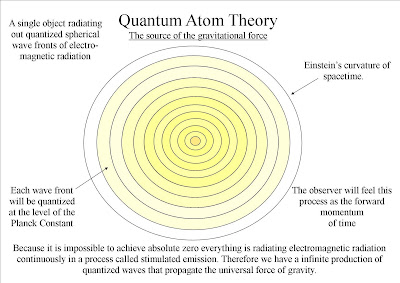Light sphere of Electromagnetic radiation create Einstein's curvature of spacetime.

~We have the inverse square law because the surface area of the light sphere increases with the square of the radius. Thus the strength of the gravitational field is inversely proportional to the square of the distance from the source.

~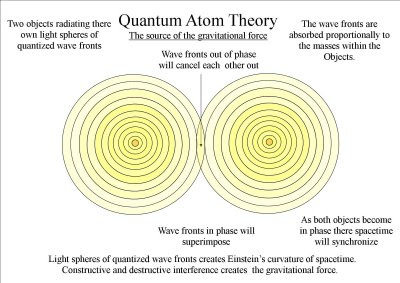The radiating energy will be entirely absorbed proportionally to the masses within the objects. This will course an unbalanced force and the two objects will resonate together.

~Wave length like x-rays can penetrate the object and therefore every single part of matter can take part in gravitational interaction.

~A quantum gravity field, as both objects become in phase there spacetime will synchronize. Time is quantized into units of radiant energy and this is why we feel the forward momentum of time moment by moment.

~